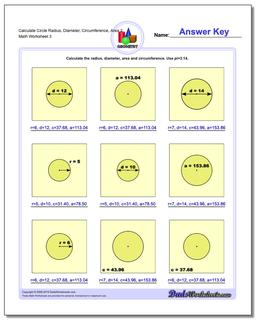# Math Worksheets: Basic Geometry: Basic Geometry: Calculate Circle Radius, Diameter, Circumference, Area 2 (Third Worksheet)## Calculate Circle Radius, Diameter, Circumference, Area 2 (Third Worksheet)

PropertyValue
DescriptionCalculate Circle Radius, Diameter, Circumference, Area 2: Calculate the radius, diameter, area and circumference given one circle fact. (Third Worksheet)
Resource TypeWorksheet